## Introduction

Research into liquids has a long history starting from the same time when the theory of gases was developed, forming the basis for our current understanding of the gas state of matter1. Yet no theory of liquid heat capacity currently exists, contrary to gases or, for that matter, solids. Consequently, liquid heat capacity is not discussed in textbooks1,2,3,4,5,6 and presents a challenge at student lectures7.

Liquids defy common approaches used to discuss the other two main states of matter, gases and solids. Interactions in a liquid are strong, therefore treating them as a small perturbation as is done in the theory of gases is not an option2. Atomic displacements in a liquid are large, therefore expanding the energy in terms of squared atomic displacements and considering the remaining terms small, as is done in the theory of solids, does not appear justified either. Strong interactions in a liquid appear to result in liquid energy being strongly dependent on the type of interactions, leading to a statement that liquid energy can not be calculated in general form2, in contrast to solids and gases where E = 3NT (kB = 1) and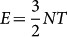at high temperature.

Previous research into liquids presents an interesting case when long-lived absence of crucial experimental data resulted in using our human experience and intuition about liquids instead. Liquids have been viewed to occupy a state intermediate between gases and solids. Liquids flow and share this fundamental property with gases rather than solids. As a result, liquids were mostly viewed as interacting gases, forming the basis for previous theoretical approaches1,2,3,4,5,6. On the other hand, Frenkel noted that the density of liquids is only slightly different from that of solids, but is vastly different from gas densities1. Frenkel has subsequently made another important proposition which has further put liquids closer to solids in terms of their physical properties. He introduced liquid relaxation time, τ, as the average time between two consecutive atomic jumps at one point in space and immediately predicted the solid-like ability of liquids to support shear waves, with the only difference that a liquid does not support all shear waves as a solid does, but only those with frequency larger than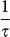because the liquid structure remains unaltered and solid-like during times shorter than τ. Frenkel's ideas did not find due support at his time, partly because of the then existing dominating school of thought advocating different views8 and consequently were not theoretically developed.

It took many years to verify Frenkel's prediction9,10,11,12,13. With the aid of powerful synchrotron radiation sources that became available fairly recently and about 50 years after Frenkel's work, it has become apparent that even low-viscous liquids maintain high-frequency modes with wavelengths close to interatomic separations13. We note that being the eigenstates of topologically disordered liquids, these modes are phonon-like in a sense that they are not entirely harmonic vibrations as in crystals.

The data from the experimental advances above, together with theoretical understanding of the phonon states in a liquid due to Frenkel, raise an important question of whether the phonon theory of liquid thermodynamics could be constructed, similar to the phonon theory of solids. Below we develop a phonon theory of thermodynamics of liquids that covers both classical and quantum regimes. We find good agreement between calculated and experimental heat capacity for many liquids in a wide temperature and pressure range.

## Results

### Theory

There are two types of atomic motion in a liquid: phonon motion that consists of one longitudinal mode and two transverse modes with frequency ω > ωF, where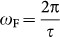is Frenkel frequency and the diffusional motion due to an atom jumping between two equilibrium positions. In turn, the phonon and diffusional motion consists of kinetic and potential parts, giving the liquid energy as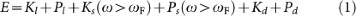where Kl and Pl are kinetic and potential components of the longitudinal phonon energy, Ks(ω > ωF) and Ps(ω > ωF) are kinetic and potential components of the energy of shear phonons with frequency ω > ωF and Kd and Pd are kinetic and potential energy of diffusing atoms. The energy of longitudinal mode is the same as in a solid, albeit different dissipation laws apply at low and high frequency1. In the next paragraph, we re-write E in the form convenient for subsequent calculations.

Pd can be omitted because Pd Ps(ω > ωF). This is because the absence of shear modes with frequency ω < ωF due to the dynamic Frenkel mechanism implies smallness of low-frequency restoring forces and therefore smallness of low-frequency potential energy of shear modes: Ps(ω < ωF) Ps(ω > ωF), where Ps(ω < ωF) is the potential energy of low-frequency shear modes14,15. Instead of low-frequency shear vibrations with potential energy Ps(ω < ωF), atoms in a liquid “slip” and undergo diffusing motions with frequency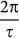and associated potential energy Pd. Pd is due to the same interatomic forces that Ps(ω < ωF), giving PdPs(ω < ωF). Combining this with Ps(ω < ωF) Ps(ω > ωF), Pd Ps(ω > ωF) follows. Re-phrasing this, were Pd large and comparable to Ps(ω > ωF), strong restoring forces at low frequency would result and lead to the existence of low-frequency vibrations instead of diffusion. We also note that because PlPs, Pd Ps(ω > ωF) gives Pd Pl, further implying that Pd can be omitted in Eq. (1).

Then, E = K + Pl + Ps(ω > ωF), where total kinetic energy in a liquid, K (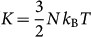in the classical case), includes both vibrational and diffusional components. E can be re-written using the virial theorem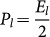and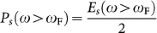(here, P and E refer to their average values) and by additionally noting that K is equal to kinetic energy of a solid and can therefore be written, using the virial theorem, as the sum of kinetic terms related to longitudinal and shear waves: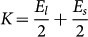, giving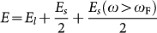. Finally noting that Es = Es(ω < ωF) + Es(ω > ωF), where the two terms refer to their solid-state values, liquid energy becomes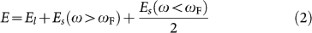Each term in Eq. (2) can be calculated using the phonon free energy,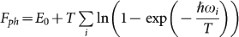, where E0 is the energy of zero-point vibrations2. In calculating the energy,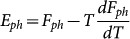, we take into account the effect of thermal expansion, important in liquids. This implies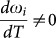, contrary to the harmonic case and gives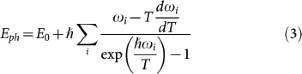Using quasi-harmonic approximation Grüneisen approximation gives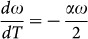, where α is the coefficient of thermal expansion16. Putting it in Eq. (3) gives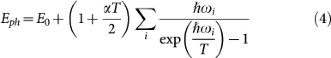The energy of one longitudinal mode, the first term in Eq. (2), can be calculated by substituting the sum in Eq. (4), Σ, with Debye vibrational density of states for longitudinal phonons,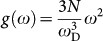, where ωD is Debye frequency. The normalization of g(ω) reflects the fact that the number of longitudinal modes is N. Integrating from 0 to ωD gives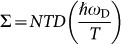, where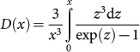is Debye function2. The energy of two shear modes with frequency ω > ωF, the second term in Eq. (2), can be similarly calculated by substituting Σ with density of states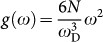, where the normalization accounts for the number of shear modes of 2N. Integrating from ωF to ωD gives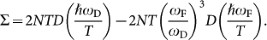. Es(ω < ωF) in the last term in Eq. (2) is obtained by integrating Σ from 0 to ωF with the same density of states, giving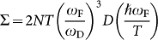. Putting all terms in Eq. (4) and then Eq. (2) gives finally the liquid energy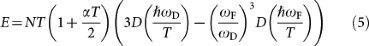where we have omitted zero-point vibration energy E0 because below we calculate the derivative of E. We note that in general, E0 is temperature-dependent because it is a function of ωF and τ (see Eq. (2)). However, this becomes important at temperatures of several K only, whereas below we deal with significantly higher temperatures.

Eq. (5) spans both classical and quantum regimes, an important feature for describing the behavior of liquids discussed below.

We note that the presented phonon theory of liquids operates at the same level of approximation as Debye theory of solids, particularly relevant for disordered isotropic systems2 such as glasses and liquids. The result for a harmonic solid follows from Eq. (5) when ωF = 0, corresponding to infinite relaxation time and α = 0.

### Comparison with experimental data

We now compare Eq. (5) to the experimental data of heat capacity. We have used the National Institute of Standards and Technology (NIST) database17 that contains cv for many liquids and have chosen monatomic nobel liquids, molecular liquids, as well as hydrogen-bonded network liquids. We have used cv measured on isobars. We aimed to check our theoretical predictions in a wide range of temperature and therefore selected the data at pressures exceeding the critical pressures of the above systems where they exist in a liquid form in the broad temperature range. As a result, the temperature range in which we calculate cv is about 100–700 K for various liquids (see Figures 1, 2, 3 and 4). Experimental cv of metallic liquids were taken from Refs.18,19 at ambient pressure. We note that previously, we have considered cv of some of these metals on the basis classical approach only14. The total number of liquids considered is 21.

We numerically evaluated Debye functions in Eq. (5) for each temperature and calculated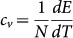. We have taken viscosity data from the NIST database at the same pressures as cv and converted it to τ using the Maxwell relationship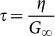, where η is viscosity and G is infinite-frequency shear modulus, giving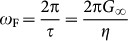. Viscosities of liquid metals and F2 were taken from Refs.20,21,22,23,24,25 and Ref.26, respectively.

We note that Debye model is not a good approximation in molecular and hydrogen-bonded systems where the frequency of intra-molecular vibrations considerably exceeds the rest of frequencies in the system (e.g. 2260 K in O2, 3340 K in N2, 3572 K in CO). However, the intra-molecular modes are not excited in the temperature range of experimental cv (see Figures 34). Therefore, the contribution of intra-molecular motion to cv is purely rotational, crot. The rotational motion is excited in the considered temperature range and is therefore classical, giving crot = R for linear molecules such as CO, F2, N2 and O2 and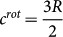for molecules with three rotation axes such as CH4, H2S, H2O and D2O. Consequently, cv for molecular liquids shown in Figures 34 correspond to heat capacities per molecule, with crot subtracted from the experimental data. In this case, N in Eq. (5) refers to the number of molecules.

We also note that in H2O, approximately half of experimental cv is the configurational contribution due to the temperature-induced structural modification of the hydrogen-bonded network, although the precise value of this contribution is not currently settled27,28. The structural modification includes changing coordinations of H2O molecules during the continuous transition between the low-density and high-density liquid in the wide temperature range28. Similarly to the phonon theory of solids, effects of structural changes are not accounted for in the general theory presented here. Therefore, experimental cv for H2O and D2O shows cv with half subtracted from their values due to the configurational term and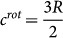subtracted further as discussed above. Due to the approximate way in which the configurational contribution is treated for H2O and D2O, the agreement between the experimental and calculated cv should be viewed as qualitative, in contrast to the quantitative agreement for the rest of liquids considered. As for other molecular liquids above, the resulting cv represents heat capacity per molecule.

Experimental and calculated cv for 21 noble, metallic, molecular and hydrogen-bonded network liquids are shown in Figures 14. Figures 14 make one of the central points of our paper: the proposed phonon theory of liquids gives good agreement with experimental data. Importantly, calculated cv has no free adjustable parameters, but depends on ωD, α and G (see Eq. (5)) which are fixed by system properties. ωD, α and G that give the best agreement in Figures 14 are close to their typical values.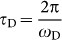used in the calculation (see the captions in Figures 14) are consistent with the known values that are typically in the 0.1–1 ps range11,29. For monatomic liquids, τD were taken as experimental τD in corresponding solids. Similarly, the difference between experimental α and α used in the calculation is within 30% on average. Finally, G used in the calculation are on the order of GPa typically measured11,29.

## Discussion

We first note that some of the considered liquids are in the quantum regime at low temperature. Taking T as the lowest temperature in Figures 14 and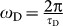, where τD are given in the captions of Figures 14, we find that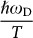is in the range 0.1–3 for various liquids. Consequently, some liquids can be described by classical approximation fairly well, whereas others require quantum approach: for example,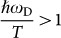for Ne, O2, N2, F2, CH4 and CO, implying progressive phonon excitation with temperature increase.

We observe that cv decreases with temperature in Figures 14. There are two main competing effects that contribute to cv in Eq. (5). First, temperature increase results in the increase of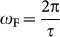. Consequently, cv decreases as a result of the decreasing number of shear waves that contribute to liquid energy and cv. Second, cv increases with temperature due to progressive phonon excitation as discussed above. The first effect dominates in the considered temperature range and the net effect is the decrease of cv with temperature seen in Figure 1.

We further observe that cv changes from approximately 3 to 2, showing a universal trend across a wide range of liquids. This can be understood by noting that at low temperature,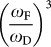and the second term in the last bracket in Eq. (5) are small, giving cv close to 3 (including the contribution from the anharmonic term). At high temperature when ωF ≈ ωD and Debye functions are close to 1, the term in the second bracket in Eq. (5) is 2, giving cv ≈ 2.

We note that on further temperature increase, cv decreases from 2 to its gas value of 1.517. This is related to the progressive disappearance of longitudinal phonons, an effect discussed in our forthcoming paper.

The decrease of cv seen in Figures 14 does not need to be generic. Indeed, when the phonon excitation dominates at low temperature, as in the case of strongly quantum liquids such as H2 or He, cv increases with temperature17.

Good agreement between theoretical and calculated cv makes several important points. First, it is interesting to revisit the statement that liquid energy can not be calculated in general form because interactions are both strong and system-specific2. In the proposed phonon theory of liquids, this difficulty does not arise because strong interactions are treated from the outset as in the theory of solids.

Second, the proposed phonon theory of liquids has an advantage over the previous approach where liquid potential energy is calculated from correlation functions and interatomic interactions2,3. Starting from the earlier proposals30, this approach was developed in several directions (see, e.g., Refs.2,3,31,32,33,34) and can be used to calculate the energy of simple liquids where interactions are pair and short-ranged and correlations are two-body as is the case in, for example, noble liquids or hard-sphere models. On the other hand, the calculations become intractable in general case3,33, particularly when interactions and correlations become complex (e.g., many-body) as in the liquids discussed above, precluding the calculation of cv and, consequently, understanding and interpreting experimental data. On the other hand, if the phonon states of the liquid depend on τ only as proposed by Frenkel1, the liquid energy and cv depend implicitly on τ only, even though correlation functions and interatomic interactions may affect both cv and τ in a complex way. Then, the relationship between cv and τ becomes fairly simple (see Eq. (5)) and explains the experimental behavior of a wide class of liquids, both simple and complex.

The last assertion is important for a general outlook at liquids: despite their apparent complexity, understanding their thermodynamics may be easier than previously thought. Indeed, we have good understanding of solid thermodynamics based on phonons no matter how complicated interactions or structural correlations in a solid are. Our current results suggest that the same can apply to liquids.